# Chapter 8 Boltzmann's Equation and its solution

So far in our discussion of multidimensional problems we have been focussing on continuum fluids governed by partial differential equations. Despite the fact that treating fluids as continua seems entirely natural, and gives remarkably accurate representation in many cases, we know that fluids in nature are not continuous. They are made up of individual molecules. A continuum representation is expected to work well only when the molecules experience collisions on a time and space scale much shorter than those of interest to our situation. By contrast, when the collision mean-free-path is either an important part of the problem, as it is, for example, when calculating the viscosity of a fluid, or when the collision mean-free-path (or time) is long compared with the typical scales of the problem, as it is for very dilute gases and for many plasmas, a fluid treatment cannot cope. We then need to represent the discrete molecular nature of the substance as well as its collective behavior.
Even so, it is unrealistic in most problems to suppose that we can follow the detailed dynamics of each individual molecule. There are p/kT=105(Pa)/[1.38×10−23(J/K)×273( K)]=2.65×1025 molecules, for example, in a cubic meter of gas at atmospheric pressure and 0 degrees C temperature (STP). Even computers of the distant future are not going to track every particle in such an assembly. Instead a statistical description is used. The treatment is common to many different types of particles. The particles under consideration might be neutrons in a fission reactor, neutral molecules of a gas, electrons of a plasma, and so on.

## 8.1  The Distribution Function

Consider a volume element small compared with the size of the problem but still large enough to contain very many particles. The element is cuboidal d3x=dx.dy.dz with sides dx, see Fig. 8.1. It is located at the position x. We want a sufficient description of the average properties of the particles in this element.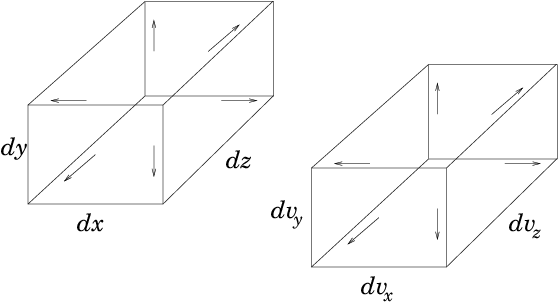Figure 8.1: The phase-space element is six-dimensional and selects particles that lie in a space element d3x and simultaneously in a velocity element d3v.
We use a statistical description originally invented by James Clerk Maxwell called the "distribution function". The distribution function is a quantity f(v,x,t) that is a function of velocity v, position x, and time t. The distribution function is defined by considering an element in velocity-space d3v=dvx.dvy.dvz with sides dv located at velocity v. Any particle whose velocity components lie simultaneously in the ranges vx→vx+dvx, vy→ vy+dvy, vz → vz+dvz, is inside that velocity element.
Then at any time t the distribution function f(v,x,t), is such that the number of particles in the spatial element d3x that have velocities in the velocity element d3v is
 f(v,x,t) d3v d3x.
(8.1)
The distribution function is therefore the density of particles in the six-dimensional "phase-space" combining velocity and space. Its utility arises from the presumption that because of the enormous number of particles in the problem we can let the velocity and spatial elements, that is the phase-space element d3vd3x, become almost infinitesimally small and yet still have a large number of particles in it. With a large number of particles, statistical descriptions make sense. In particular, it makes sense to think of f as a kind of continuous fluid in six dimensions. Obviously if the phase-space element is shrunk down to a sufficiently small size, then eventually there will be very few particles in it. The discreteness of the particles becomes visible and eventually there are either one or no particles in each tiny volume. But if we can shrink the element enough that it is small compared with the smallest scales in the problem while it still contains a large number of particles, then we have a sufficient statistical description if we know f everywhere, but we don't know the coordinates of each individual particle. The most famous of all such distribution functions is the Maxwellian, which is
 f(v,x,t) = n(x,t) ⎛⎝ m 2πk T ⎞⎠ 3/2 exp ⎛⎝ − mv2 2kT ⎞⎠ .
(8.2)
Here m is the mass of the particles, T their temperature, and k Boltzmann's constant. The squared velocity v2=v.v=vx2+vy2+vz2 appearing in the exponential makes the distributions in the different coordinate directions separable
 exp ⎛⎝ − mv2 2kT ⎞⎠ =exp ⎛⎝ − mvx2 2kT ⎞⎠ exp ⎛⎝ − mvy2 2kT ⎞⎠ exp ⎛⎝ − mvz2 2kT ⎞⎠ .
(8.3)
See Fig. 8.2.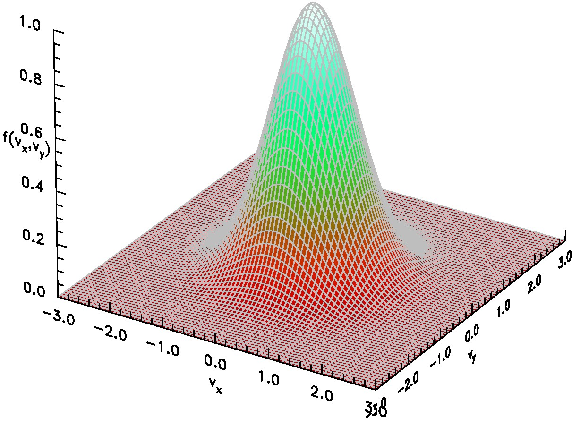Figure 8.2: A Maxwellian distribution function in two dimensions displayed as a perspective view of the surface f(vx,vy), with velocities (vx,vy) normalized to the thermal velocity
 √ 2T/m

. This can be considered to be the proportional to the distribution at a fixed value of vz, since the Maxwellian is separable.
The factor
 ⎛⎝ m 2πkT ⎞⎠ 3/2

normalizes the distributions in the three velocity dimensions. It is equal to the inverse of the integral of eq. (8.3) over all velocities. Therefore the leading term n(x,t) is just the density in space (not phase-space). It might vary with position or time. The Maxwellian distribution is what occurs in thermodynamic equilibrium when there are no substantial effects driving the velocity distribution away from its natural form. But there are many important situations where non-thermal, that is non-Maxwellian, distributions arise.
The distribution function directly determines the mean flow velocity, and for particles whose internal energy is unimportant, the energy density. The particle flux density, which is the fluid velocity times the fluid density, is
 Γ = n v = ⌠⌡ v f(v,x,t) d3v.
(8.4)
The kinetic energy density, which for a stationary fluid can be considered the density times 3/2 times the temperature is
 E = 3 2 n k T = ⌠⌡ 1 2 m v2 f d3v.
(8.5)
When the distribution in a specific coordinate direction does not concern us, perhaps because it is known, or because by symmetry it is unimportant, we often reduce the number of dimensions which we track. For example, often we might address only the x-direction velocity, vx. In that case, we use a one-dimensional distribution function,
 fx(vx) = ⌠⌡ f(v) dvydvz,
(8.6)
that is the integral of the full three dimensional distribution over the ignorable velocity coordinates. In effect, fx(vx) picks out a particular vx but includes all possible vy and vz. So the number of particles in the velocity element dvx is fx(vx)dvx.
The distribution function first arose in connection with the kinetic theory of gases. Its use is therefore often referred to as "kinetic theory".

## 8.2  Conservation of Particles in Phase-Space

Boltzmann's equation governs the conservation of particles; not just in space, which is the continuity equation (4.5), but in phase-space. When solved, it tells us what the distribution function actually is. Its derivation is mathematically very much like the derivation of the fluid continuity equation. The main complication is that one needs to think in six dimensions! We'll usually illustrate this thinking in a (2-dimensional) diagram, using just one space (x as the abscissa) and one velocity (v as the ordinate) dimension. See Fig. 8.3.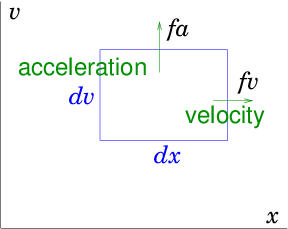Figure 8.3: In phase-space, velocity v carries a particle in the x-direction, acceleration carries it in the v-direction. Particle flux out of an element dvdx arises from the divergence of the fluxes fv and fa in the respective directions
As time passes, particles move in phase-space. The rate of change of x is the velocity dx/dt=v. The rate of change of velocity v is acceleration dv/dt=a. Generally acceleration arises from force (per particle) divided by particle mass. The force might be gravity, or (for charged particles) electric or magnetic field. An individual particle thus moves through the phase-space (in the xv plane of our diagram). If we therefore consider some phase-space volume we can write the conservation of particles within it just as we did for the fluid continuity equation as
 ∂f ∂t + ∇ps.(f vps) = ∂f ∂t + ∂ ∂x .(f v) + ∂ ∂v . (f a) = S .
(8.7)
Here vps is the "phase-space velocity", a six-dimensional vector consisting of the combination of the velocity in space and the acceleration. And ∇ps is the gradient operator in phase space, likewise a six-dimensional vector.
vps=
v
a

=

 vx
 vy
 vz
 ax
 ay
 az

and  ∇ps=

v

=

 ∂ ∂x

 ∂ ∂v

=

 ∂/∂x
 ∂/∂y
 ∂/∂z
 ∂/∂vx
 ∂/∂vy
 ∂/∂vz

.
(8.8)
The notation most usually used is to write out the space and velocity parts of the derivatives separately.
 ∂ ∂x . (fv) = ∂(f vx) ∂x + ∂(f vy) ∂y + ∂(f vz) ∂z
(8.9)
and
 ∂ ∂v . (f a) = ∂(f ax) ∂vx + ∂(f ay) ∂vy + ∂(f az) ∂vz .
(8.10)
This helps us remember we are dealing with phase-space. Equation (8.7) expresses the fact that the rate of change of number particles in a phase-space element is equal to the rate at which they are flowing inward across its boundary plus the combined source rate inside the element, S (all per unit volume). Particles flow across the boundary either by moving in space across the boundary of d3x, or by accelerating (moving in velocity-space) across the boundary of d3v.
A final simplification arises in eq. (8.7) because of what a partial derivative means. It means take the derivative keeping all the other phase-space coordinates constant. In other words, the partial x-derivative holds y,z,vx,vy,vz constant. The partial derivative of any vj with respect to any xk is therefore zero, which means that in the spatial divergence eq. (8.9) the velocity factors can be taken outside the spatial derivatives to write
 ∂ ∂x .(f v) = v. ∂ f ∂x = vx ∂f ∂x + vy ∂f ∂y + vz ∂f ∂z .
(8.11)
That rearrangement is always possible. If the acceleration of a particle does not depend on its velocity (or depends on it in such a way that ∇v.a=0, which is the case for the Lorentz force) then we can do the same for the acceleration term.
Then we arrive at Boltzmann's equation
 ∂f ∂t + v. ∂f ∂ x + a. ∂f ∂v = S = C.
(8.12)
The source term on the right hand side of Boltzmann's equation contains not only literal creation or destruction of particles (e.g. by chemical or nuclear reactions), but also any instantaneous changes of velocity, in other words collisions. A collision that does not destroy or create a particle of the type we are tracking can nevertheless change its velocity abruptly45. This change in velocity immediately transports the particle from one velocity to another. The particle jumps to a different position in phase-space. That constitutes a "sink" at the old velocity and a "source" at the new velocity. Chemical or nuclear reactions also occur as a result of collisions, of course. Consequently essentially all the phenomena that contribute to the Boltzmann equation's source (with the exception of spontaneous - e.g. radioactive - decay of the particles) are collisions; and the source term is usually called the "collision" term and written C instead of S.
The collision term C(v,x,t) is the rate per unit phase-space-volume of generation (or removal if it is negative) of particles at position x having velocity v. It naturally depends also upon the distribution function f itself. For example the rate per unit volume at which collisions occur removing particles of a certain velocity is proportional to the number of such particles present in the first place.

## 8.3  Solving the Hyperbolic Boltzmann Equation

### 8.3.1  Integration along orbits

If we know the collision term C, as well as a, then clearly Boltzmann's equation is a first-order linear partial differential equation (in seven total dimensions including time, or less if there are ignorable coordinates). Since it is first order linear with a single scalar dependent variable46, f, it is hyperbolic. That means we may solve it as an initial value problem.
The most natural way to do this is to follow particle trajectories in phase-space, which we will call particle orbits. Any individual particle moves in accordance with
 d x d t = v, d v d t = a;       i.e. d d t ⎛⎝ x v ⎞⎠ = ⎛⎝ v a ⎞⎠
(8.13)
This is an ordinary differential equation, which we know how to solve (assuming a is known), starting from some initial position in phase-space x0, v0. But how does this orbit help us to solve Boltzmann's equation for the distribution function? It helps us because Boltzmann's equation is an equation for the rate of change of f moving along a particle phase-space orbit.
Suppose we catch a six-dimensional ride on one of the particles and move with it, looking out into the phase-space near to us and measuring the particle density there; watching it change as time goes by. The rate of change of the distribution function will be precisely that given by the left hand side of Boltzmann's equation. See Fig. 8.4 for a visualization.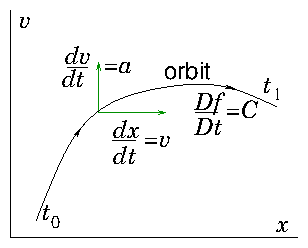Figure 8.4: A phase-space orbit is determined by a first order ordinary differential equation. The Boltzmann equation states that the rate of change of the distribution function along phase-space orbits is equal to the collision term.
First let's convince ourselves that is true. If, during our ride, we measure the difference in f between times different by a small interval dt. The f will be different because (1) it may have intrinsic variation with time, resulting in a change dt ∂f/ ∂t; (2) it may have variation with space so our motion has carried us a distance dt v, to a place where f is different by dt v.∂f/∂x; or (3) it may have variation with velocity so our motion in velocity space (acceleration) has carried us a velocity-"distance", dt a, to where f is different by dt a.∂f/∂v. The total of these three, divided by dt, is the rate of change of f along the orbit. That's the left hand side of eq. (8.12).
Second, why is this identity with the total derivative the case? It is because the flow in phase-space is divergenceless, ∇ps.vps=0. Just as for an ordinary three-dimensional fluid for which ∇.(ρv) = v.∇ρ if ∇.v=0, implying Dρ/Dt=S, similarly for a dimensionless flow in phase-space. The phase-space flow is divergenceless if the acceleration has the requisite property ∇v.a=0. This can be interpreted as a statement that there is no dissipation.
Third, how does this identity help us? It reduces Boltzmann's equation to an ordinary differential equation along the orbits. Writing the total differential as D/Dt it becomes
 ∂f ∂t + v. ∂f ∂ x + a. ∂f ∂v = Df Dt = C.
(8.14)
We can integrate the second equality immediately to obtain:
 f(v1,x1,t1) − f(v0,x0,t0) = ⌠⌡ 1 0 C dt.
(8.15)
The integral is along the orbit, whatever that might be in phase space, from the initial position (0) to the final position (1). The final value of the distribution function, measured at the final values of velocity, position, and time, is equal to the initial value of the distribution function at the initial values of velocity, position, and time, plus the integral of the collision term along the orbit. The easiest case to deal with is if there are no collisions, C=0. Then the initial and final distribution functions are equal in value. A fact we express by saying, in the absence of collisions, the distribution function is "constant along orbits".
It is vital to realize that constant along orbits generally does not mean that the distribution function is the same function of velocity at 1 as it was at 0. No, the orbital velocity has changed between those positions. So although f has the same height, that height does not occur at the same velocity. Fig. 8.5 illustrates this fact.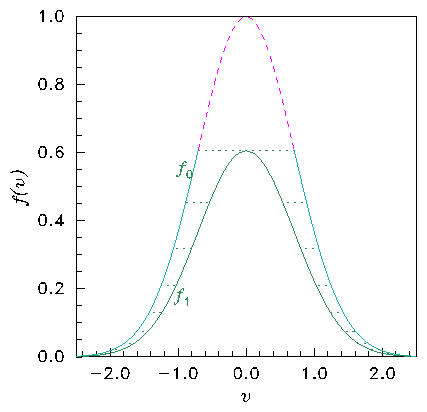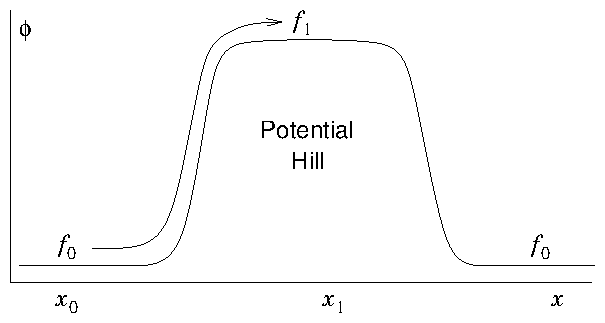Figure 8.5: In the collisionless Boltzmann equation the distribution is constant along orbits. The distribution (a) is different at the top of a potential hill (b) because the speed on an orbit is smaller (conserving energy). The distribution values f0=f(x0) and f1=f(x1) are the same but at different velocities. Orbits have moved the distribution along the horizontal dotted lines in (a). The lowest velocity orbits of distribution f0 (upper dashed part) can't reach the top of the hill where f1 is, and do not contribute to it.

### 8.3.2  Orbits are Characteristics

Every hyperbolic partial differential equation can be analysed in a manner equivalent to the integration along orbits. This approach is called the method of characteristics. The terminology "characteristic" is the general term for what we've called in the context of the Boltzmann equation an "orbit". Suppose we have a first order linear equation, an advection equation with source
 v. ∂ ∂x ψ = S,
(8.16)
in which the components of the N-dimensional vector v are simply known functions of ψ and the N-dimensional independent variables x. Introduce a new parameter t which is going to serve like time. (If the original equation already contained time as one of the independent variables, treat it just as one component of the vector v and use the new t as a parameterization.) Think of the v as velocities in N-dimensional space such that dx/dt=v. Remember, in the original formulation, those v were just the coefficients of the partial derivatives in the respective directions. What we've done is to address the question "what if the coefficients v were velocities?" in respect of the new t parameter we introduced. The answer is that starting from any point x we would move with the velocity v and thereby trace out an orbit in the x-space. This orbit is what is called the characteristic of the differential equation. As we follow the characteristic, the equation we would be satisfying would be
 v. ∂ ∂x ψ = d x dt . ∂ ∂x ψ = N∑ j=1 d xj dt . ∂ ∂xj ψ = d ψ dt ⎢⎢ orbit =S.
(8.17)
This equation is an ordinary differential equation along the characteristic and can be integrated as ψ1−ψ0 = ∫01 Sdt. This process is exactly what we did for Boltzmann's equation. The only difference is that Boltzmann's equation already contained time. Fortunately the coefficient of
 ∂ ∂t

in Boltzmann's equation is 1. Therefore it was possible to choose the time-like parameter to be actual physical time, which we did. We could however have made a different choice, if we'd preferred. We also used notation familiar from fluid theory for the "convective" derivative D/Dt, but that is no different from d/dt along the orbit.
Higher order scalar equations can be rendered into first order, vector (multiple dependent variable) equations, as we saw before. If they are hyperbolic, then they have characteristics which correspond to the coefficients of the eigenvectors of the equations. Those eigenvectors must be real otherwise the presumption that there are real characteristics breaks down. The condition, therefore, for a system of vector equations to be hyperbolic is that they are diagonalizable with real eigenvectors.

## 8.4  Collision Term

The importance of the Boltzmann equation's collision term depends upon the application. In some plasma and gravitational applications it can be completely neglected and ignored. Collisions are nothing. Then the equation of interest is called the Vlasov equation.
 ∂f ∂t + v. ∂f ∂ x + a. ∂f ∂v = 0.
(8.18)
At the other extreme, when applied forces are negligible so a=0, and the problem is homogeneous and steady state ∂/∂x=0, ∂/∂t=0, all that is left of the Boltzmann equation is C=0. So then collisions are everything!
Also, the form of the collision term depends upon application. Especially it depends upon whether the important collisions are with the same particles or with some particles of a different type that are described by a different distribution function.

### 8.4.1  Self-scattering

Self-scattering dominates, for example, a simple unreactive monatomic gas. There is just one species of particle. And elastic self-scattering is the only type of collision present. Then the integrals over all velocity space of C, vC, and v2C are zero. That is a simple consequence of particle, momentum, and energy conservation. With self-scattering, though, other complications are severe. The rate at which collisions take place depends upon a product f(v1)f(v2) of the distribution functions of the two colliding particles of different velocities (so it is non-linear). It is multiplied by the collision rate, which is the cross-section (a function of relative speed) times the relative speed σ|v1v2|. It is then integrated over the velocity v2 of the target particle (so Boltzmann's equation becomes an integro-differential equation). Generally substantial approximation is necessary to make the collision term managable, even for numerical solution.

### 8.4.2  No self-scattering

If, however, the dominant interactions are with a different type of particle, then momentum and energy of the first species is not necessarily conserved. It can be transferred to the second species. But at least the collision term is linear in f, and if the initial velocity distribution of the second species is known or may be neglected, then substantial reduction of the need for integration can occur.
For example, as illustrated in Fig. 8.6, a form of collision term that approximately represents charge-exchange collisions at a fixed rate ν between singly charged ions (the species whose Boltzman equation we are trying to solve) and neutrals of the same element (the target species 2) is
 C(f) = −νf(v) +νf2(v).
(8.19)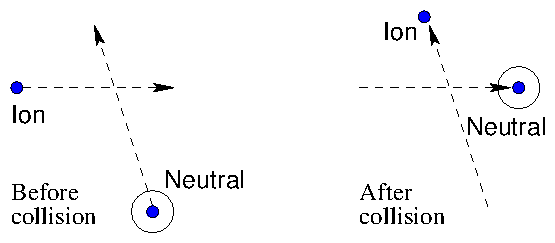Figure 8.6: Charge exchange collisions, where an electron is transferred from a neutral to an ion, give rise to a simple collision term. If they occur at a constant rate, ν, eq.  8.19 applies.
This is sometimes called the BGK collision form. It represents depletion of the original ions at rate ν giving the term −νf(v), and their direct replacement at the same rate by new ions. The newly born ions, before their collision, were neutrals. They retain the velocity distribution f2(v) they had before the collision, because the collision just transfers an electron from one to the other.
Another idealized example (Fig. 8.7) is when collisions are with heavy stationary targets (which therefore acquire negligible recoil energy) which happen to scatter equally, isotropically, in all directions.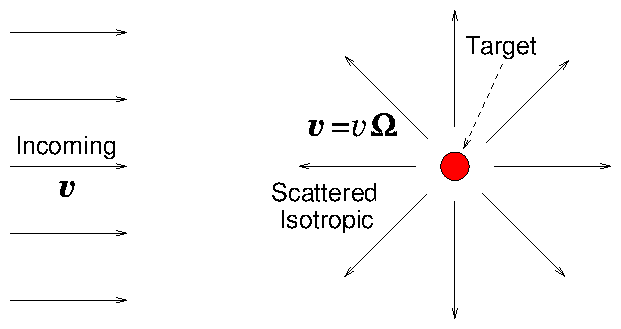Figure 8.7: Isotropic scattering (an idealized approximation) gives particles emerging equally in all directions Ω. With heavy targets, v is not changed in magnitude, only in direction. Eq.  (8.20) is the result.
In a collision, a particle just changes the direction of its velocity, not its magnitude. If the density of targets is n2 and the collision cross-section is σ, then
 C(f) = −n2 σv ⎛⎝ f(v) − ⌠⌡ f(v)d2Ω/4π ⎞⎠ .
(8.20)
Here d2Ω = sinθdθdχ is the element of solid angle, and the integral is over the angular position (θ,χ) on the surface of the sphere in velocity space at constant total velocity v. In other words, the second term is the average of the distribution function over all directions, at v. This type of collision scatters the velocity direction, thus tending to remove any anisotropy (variation with angles θ or χ.)
It should be noticed that in these examples where self-collisions can be ignored, the collision term generally consists of two parts. The first is negative, the removal or "sink" rate of particles that collide with whatever targets happen to be present (−n2σv f in eq.8.20). The second is positive, the "source" rate of particles from all mechanisms. When non-reactive gases are being treated, the source is only the re-emergence of particles from collisions. But in other situations, such as neutron transport in a reactor, generation of new particles from reactions or spontaneous emission from the target medium may be equally important.
For multiple target species, j, the sink term is the sum of collisions with all target types. And this is often written in shorthand as −Σt×( v f), with
 Σt = ∑ j nj σj;
(8.21)
and referred to in reactor physics literature as the "macroscopic cross-section". This terminology is unfortunate because the quantity Σt has units m−1 not m2, and is an inverse attenuation-length, not a cross section. When the targets are stationary, Σt is isotropic: the rate of collisions is independent of the direction of particle velocity. The source term, by contrast, is not usually isotropic because it includes the emergence of particles from pure scattering events. Scattering, even from stationary targets, usually partially retains any anisotropy in the distribution function itself. (The conditions of eq. 8.20 are a non-typical idealization.)

## Worked Example: Solving Vlasov's Equation

Consider a steady-state situation, one dimensional in space and velocity, where acceleration arises only from a spatially varying potential energy of the form ϕ(x)=ϕ0exp(−x2/w2), so a=−1/mdϕ/dx, and collisions are negligible. If the distribution function at |x|→ ∞ is equal to f(v) = exp(−m v2/2T), and ϕ0 ≥ 0, find the distribution function f(v,x) for all x, and v. Can you solve this problem if ϕ0 < 0?

The steady collisionless one-dimensional Boltzmann (Vlasov) equation is
 0= D f D t = v. ∂f ∂ x + a. ∂f ∂v
(8.22)
The equations of the orbit (the characteristics) are
 d x d t = v; d v d t =a=− 1 m dϕ d x .
(8.23)
Multiplying the second of these by the first we find
 v d v d t + 1 m dϕ d x d x d t =0,
(8.24)
which may be immediately integrated to find
 1 2 m v2 + ϕ = const.
(8.25)
We have derived the conservation of energy, kinetic plus potential. The constant can be considered to be the kinetic energy at infinity, 1/2mv2, where the potential (ϕ) is zero.
For Vlasov's equation, the distribution function, f is constant along the orbits. Therefore
 f(v,x) = f∞(v∞) = exp(−m v∞2/2T) = exp(−[m v2+2ϕ(x)]/2T).
(8.26)
Substituting for ϕ(x) we find
 f(v,x) = exp(−[m v2+2ϕ0 e−x2/w2]/2T) = exp(− m v2 2T )exp(− ϕ0 T e−x2/w2).
(8.27)
If ϕ0 > 0, then no matter how small v2 is, there is a real solution for v to the conservation equation 1/2 m v2+ ϕ = 1/2 m v2. Therefore this expression for f is valid for all v. The distribution is everywhere Maxwellian, but its density varies with position. However, if ϕ0 < 0 then, everywhere that ϕ is negative, there is a minimum speed
 √ −2ϕ/m

, below which there is no real solution for v. These are the trapped orbits. They do not extend to infinity, but are reflected because they reside in the potential well. The value of f on those trapped orbits is undefined by the boundary condition at infinity, and must be determined otherwise, e.g. from the initial conditions. Fig. 8.8 shows an example solution.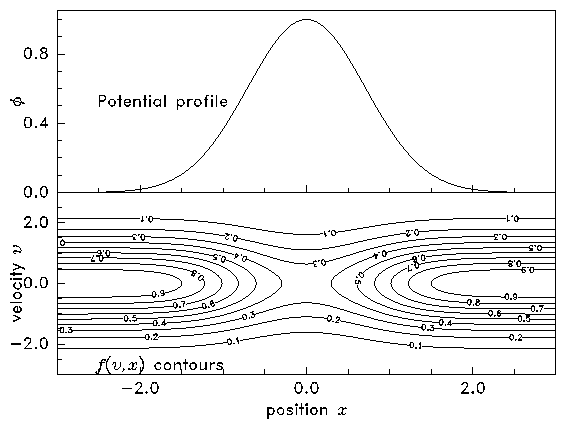(a)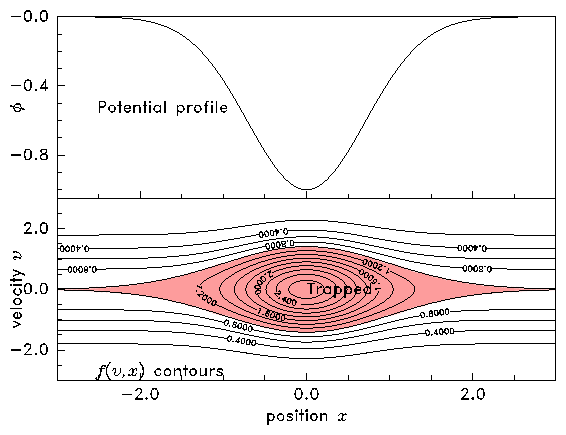(b)
Figure 8.8: Contours of constant f(v,x) are also orbits. Therefore the orbits can be plotted simply by contouring f, whose value is determined by the total (kinetic plus potential) energy at any point in phase-space. When the potential has a hill (a), all orbits extend to x→ ∞ and f is determined by boundary conditions. When the potential has a well (b), the value of f on trapped orbits (shaded) is undetermined. [The parameters used in these plots are ϕ0/m=±1, w=1, T/m=1.]

## Exercise 8. Boltzmann's Equation.

1. Divergence of acceleration in phase-space.
(a) Prove that particles of charge q moving in a magnetic field B and hence subject to a force qv×B, nevertheless have ∇v.a=0.
(b) Consider a frictional force that slows particles down in accordance with a=−Kv, where K is a constant. What is the "velocity-divergence", of this acceleration, ∇v.a? Does this cause the distribution function f to increase or decrease as a function of time?

2. Write down the Boltzmann equation governing the distribution function of neutral particles of mass m in a gravitational field
 g ^ x

, moving through matter that consists of two different species of density na, nb whose only effects are: species a absorbs the particles with a cross-section σa independent of speed; species b emits the particles with a Maxwellian distribution of small temperature Tb, by radioactive decay with a half-life tb.
Solve the equation (analytically) in uniform steady state (
 ∂ ∂t = ∂ ∂x =0

), in the limit kTb << m g/naσa, to find the distribution function fx(vx).